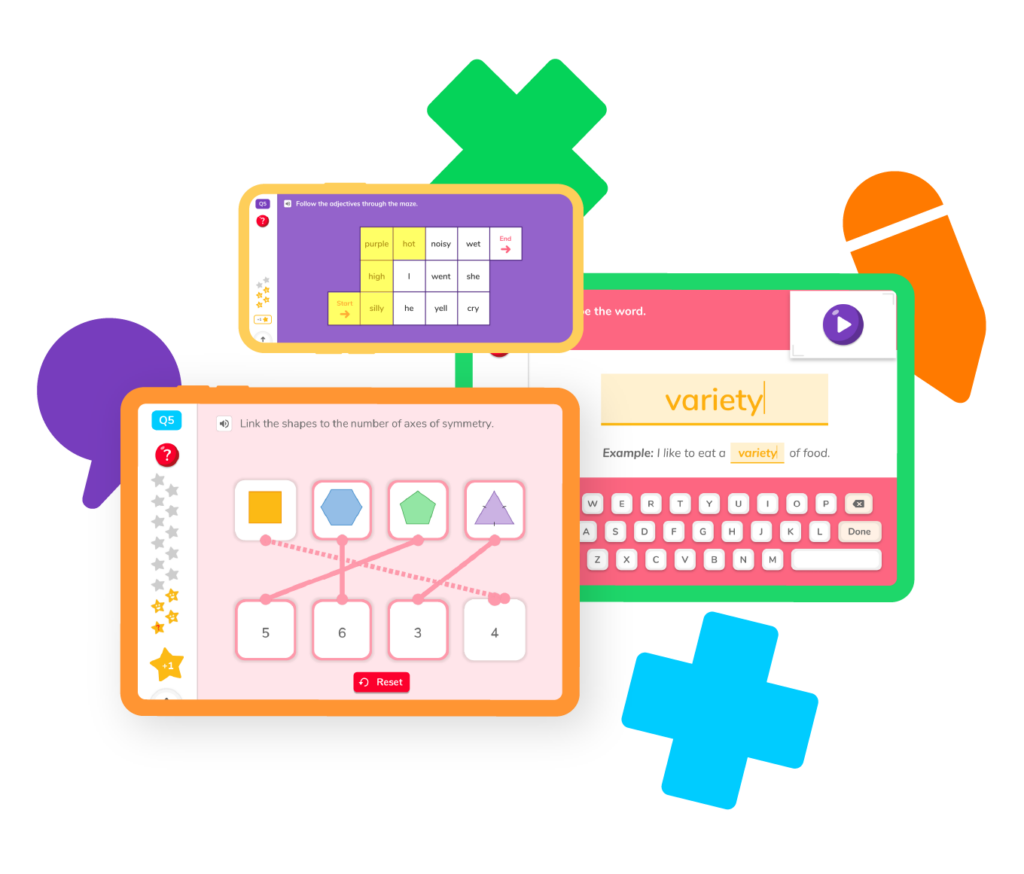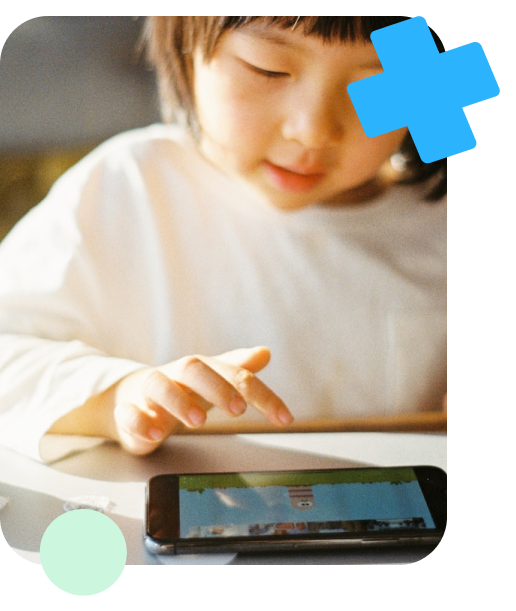# Learning Multiplication

Did you know whenever we want to count the same number multiple times quickly, we can multiply? For that reason, multiplication is often referred to as the speedy form of addition. We multiply when counting groups of items, finding the area of different shapes, and calculating money. This makes multiplying a principal skill to learn in math and one we want to master!## Learning MultiplicationMultiplication is introduced in math as early as third grade when your child will begin practicing their first multiplication facts. By fourth grade, your child is taught the parts of a multiplication problem and how to use a multiplication chart, multiply two and three-digit numbers with long multiplication, and the different properties of multiplication

As your student progresses to fifth-grade math, your student will learn the distributive and identity properties of multiplication. This will help them see new ways to solve math problems and increase their likelihood of getting the answers quicker and easier.

### When to Start Practicing Multiplication with My Child?

You may be wondering, when should I start practicing multiplication with my child? Although certain multiplication math concepts are taught in different grade levels, there is no limit to when your child can begin practicing their multiplication. If they are ready, the earlier they begin practicing the better!

## Multiplication ResourcesPracticing to become a skilled multiplier can be fun with Doodle Learning! Begin by choosing a topic about multiplication. You will discover many multiplication practice problems and learn some of the best multiplication tips and tricks from some of the very best teachers and tutors.

## What is a product?

Learn the basic parts of a multiplication problem and how to find the product.

## How to use a multiplication chart

Learn the features of a multiplication chart and tips and tricks on how to use it to find the product.

## How to do long multiplication

Practice the steps to multiplying two and three-digit numbers by long multiplication.

## How to multiply fractions

Learn how to multiply fractions with our step-by-step tips and practice questions

## Distributive property of multiplication

Explore the steps to using the distributive property of multiplication.

## Identify property of multiplication

Learn and practice the identity property of multiplication and discover how it can help you solve problems more easily!

## What is a product?

Learn the basic parts of a multiplication problem and how to find the product.

## How to use a multiplication chart

Learn the features of a multiplication chart and tips and tricks on how to use it to find the product.

## How to do long multiplication

Practice the steps to multiplying two and three-digit numbers by long multiplication.

## Multiplying fractions

Learn how to multiply fractions with our step-by-step tips

## Distributive property of multiplication

Explore the steps to using the distributive property of multiplication.

## Identity property multiplication

Learn and practice the identity property of multiplication and discover how it can help you solve problems more easily!### What is Multiplication?

Multiplication is an operation, or action, in math that allows you to quickly count a number many times. For example, if you wanted to count the number 9 eight times, you can simply multiply 9 times 8 which equals 72.

Multiplication is also considered a shortened form of addition. For example, instead of adding 2 + 2 + 2 + 2 + 2, we can simply multiply 2 x 5 to find the answer quickly. Two is the number we are multiplying and five would be the number of times we multiply it by. What are the names of those numbers when written in a multiplication sentence

### What are the Parts of a Multiplication Problem?

It is very important to know the math vocabulary terms used in a multiplication sentence in order to solve fourth and fifth-grade math word problems.

There are three main parts of a multiplication problem. They are called the multiplicand, the multiplier, and the product. In the multiplication sentence 12 x 4 = 48, the number 12 would be the multiplicand. The number 4 is the multiplier and the number 48 is the product or answer.

### What vocabulary terms do I need to know to understand multiplication?

• The multiplicand is the number in the math sentence that you are multiplying
• The multiplier is the number that shows how many times you are counting the multiplicand by
• The product is the answer you get after multiplying. It is usually found at the end of the multiplication sentence.

### When will I use multiplication in real life?

We use multiplication almost every day whether we realize we are using it or not. Most times we do it in our heads to calculate the price of many items, to calculate the amount of time it takes to do a repetitive task each week, when we calculate the size of our garden or the area of a room, when we double the size of a recipe, or even calculate the amount of money we make in a month.

Anytime we take a number and count it many times over, we can multiply it to find the total instead of adding. How grateful we are that we have the ability to multiply. Could you imagine doing 10 x 30 just by adding? It would take a lot of brain power! Multiplying makes things easy.

### When will my child begin to learn multiplication?

Students begin learning multiplication in 3rd grade when they are introduced to their first multiplication facts. They are usually taught to memorize multiplication facts through songs and using multiplication charts.

It is good to note, that if your child is doing well with basic addition, it is never too early to start practicing the basic multiplication skills, like skip counting and practicing their times tables.

### How Can I Supplement My Child’s Learning of Multiplication?

The best way to supplement your child’s learning is through online math worksheets, games, and quizzes. This exposes your child to a variety of multiplication practice problems that they will see in third to fifth-grade math. Check out the best math app for students in grades K-5 to see the many resources it has for students and parents to practice and monitor math skills.

Multiplication in math is the way you count the same number many times quickly. That is why you will often hear multiplication called ‘times’ or ‘times tables’.

The multiplicand is the number that is being multiplied or times. It usually is the first number in a multiplication sentence. For example, in the multiplication sentence 14 x 10 = 140, the number 14 is the multiplicand.

As your child advances to different grade levels, their understanding of Geometry math concepts is broadened. Fifth-grade math has the highest level of geometry math concepts of the grades in elementary. If your child stays on track, these more challenging concepts can easily be learned and practiced.

The multiplier is the number of times the multiplicand is being times by. It is usually the second number after the multiplication symbol. For example, in the multiplication sentence 4 x 15 = 60, 15 is the multiplier because it is the number of times we will count by the number 4.

Multiplying is for everyday and everyone. The more your child practices multiplication skills, the more skilled they will become and the easier they will find it, as long as you’re practicing together with the right math tools.# Are you a parent, teacher or student?

Are you a parent or teacher?

## Hi there!

Book a chat with our team